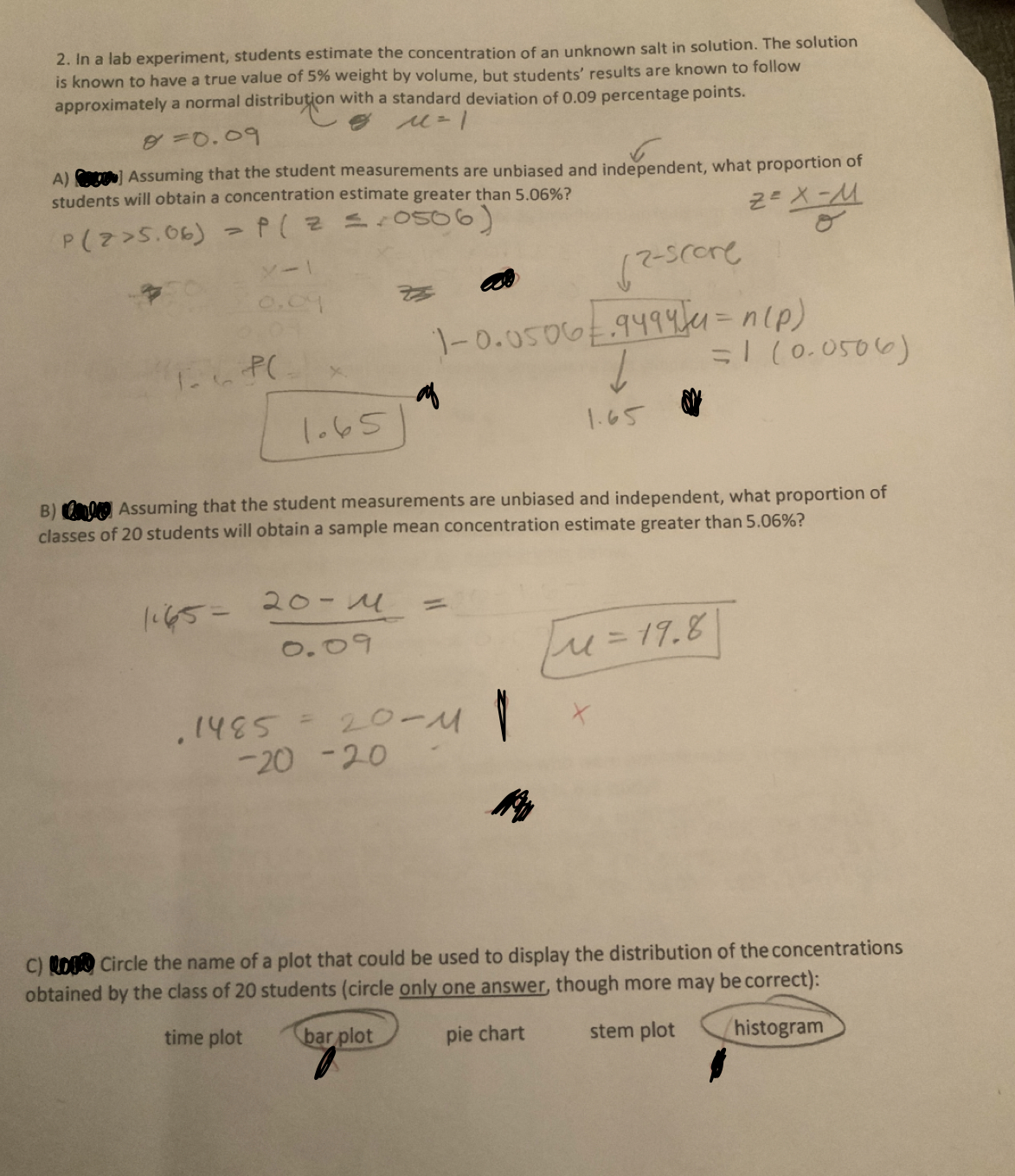# 2. In a lab experiment, students estimis known to have a true value of 5% weight by volume, but students' results are known to followapproximately a normal distribution with a standard deviation of 0.09 percentage points.ate the concentration of an unknown salt in solution. The solutionA) Assuming that the student measurements are unbiased and independent, what proportion ofstudents will obtain a concentration estimate greater than 506%?2-х-М-0.05004 npICo.oso)16SAssuming that the student measurements are unbiased and independent, what proportion ofof 20 students will obtain a sample mean concentration estimate greater than 5.06%?classes20-4o.o20 20C ts Circle the name of a plot that could be used to display the distribution of the concentrationsobtained by the class of 20 students (circle only one answer, though more may be correct):time plotpie chart stem plot histogramar plot

Questionhelp_outlineImage Transcriptionclose2. In a lab experiment, students estim is known to have a true value of 5% weight by volume, but students' results are known to follow approximately a normal distribution with a standard deviation of 0.09 percentage points. ate the concentration of an unknown salt in solution. The solution A) Assuming that the student measurements are unbiased and independent, what proportion of students will obtain a concentration estimate greater than 506%? 2-х-М -0.05004 np ICo.oso) 16S Assuming that the student measurements are unbiased and independent, what proportion of of 20 students will obtain a sample mean concentration estimate greater than 5.06%? classes 20-4 o.o 20 20 C ts Circle the name of a plot that could be used to display the distribution of the concentrations obtained by the class of 20 students (circle only one answer, though more may be correct): time plot pie chart stem plot histogram ar plot fullscreen
check_circleExpert Solution
Step 1

Let X be the concentration estimate. X follow a normal distribution with mean 5% and standard deviation 0.09 percentage points.

We need proportion of students will obtain a concentraton estimate more than 5.06% = P(X > 5.06).

Here we calculate Z score for X = 5.06 using the below shown formula. Z score is 0.67 for X = 5.06.
P(X > 5.06) = P(Z > 0.67)

Step 2

With reference to below shown Z table the the probability  value to the right of Z score 0.67 is 0.2514.

P(Z > 0.67) = P(X > 5.06) = 0.2514

Step 3

Given sample size of 20 students with mean 5% and standard deviation 0.09%. The standard deviaiton of sample will be =   σ/√n, where n is the sample size and   &sigma...

### Want to see the full answer?

See Solution

#### Want to see this answer and more?

Solutions are written by subject experts who are available 24/7. Questions are typically answered within 1 hour*

See Solution
*Response times may vary by subject and question
Tagged in

### Statistics